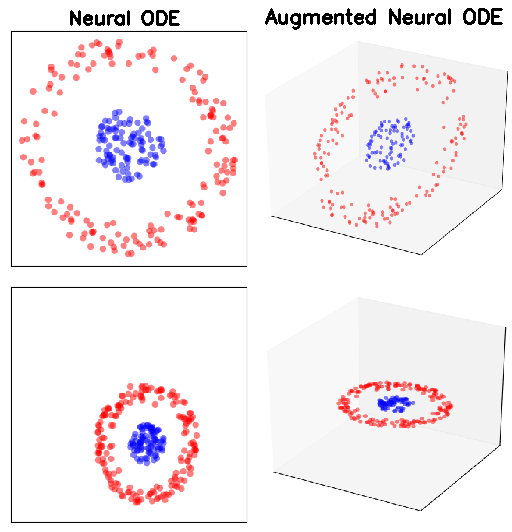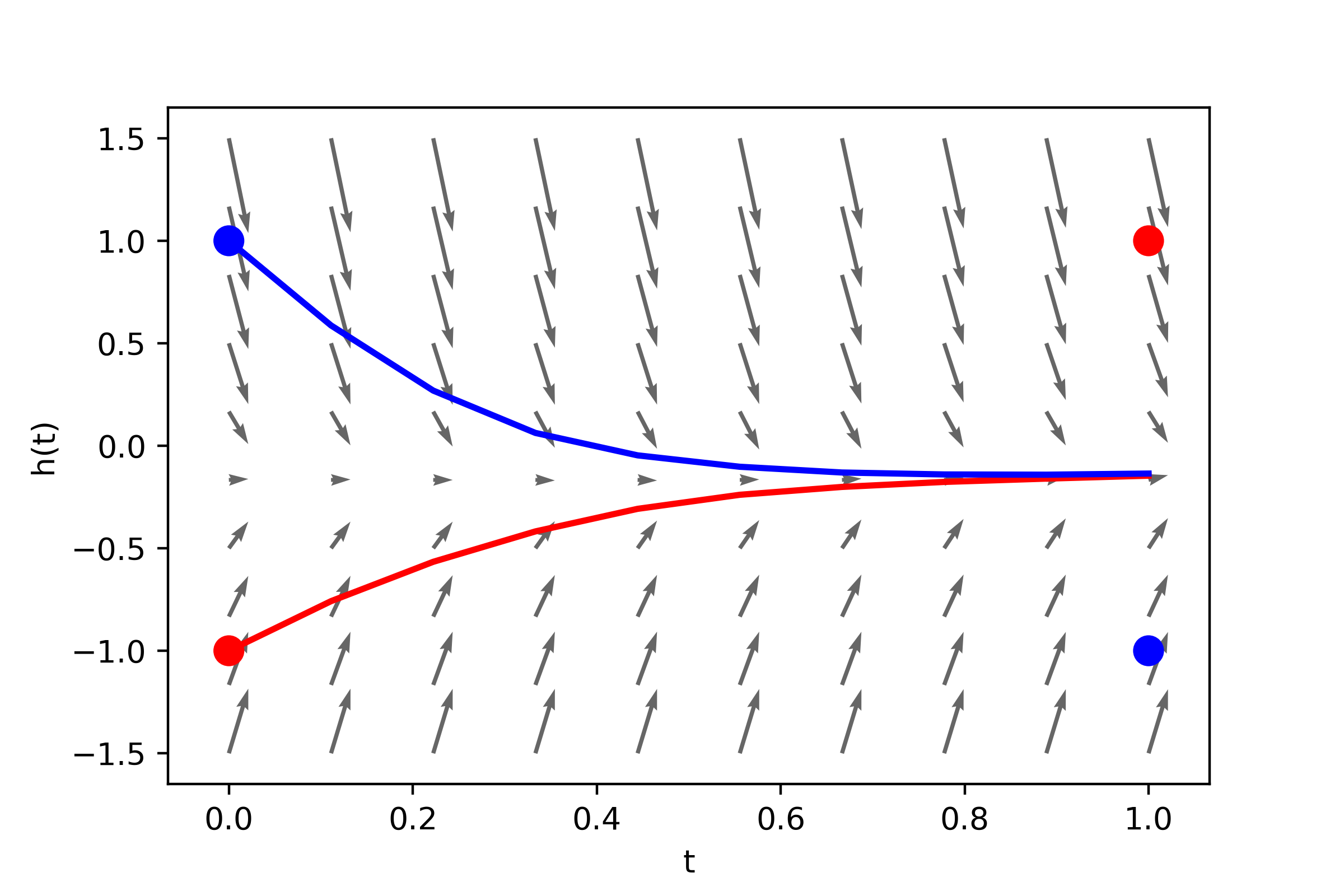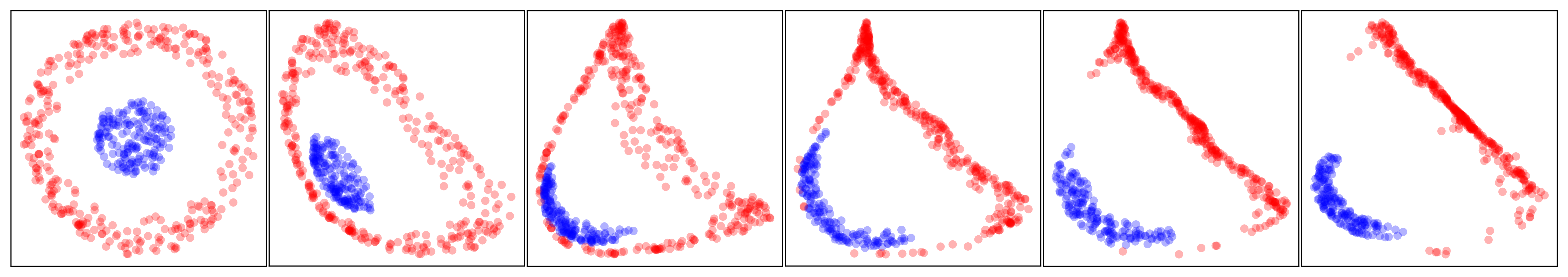/ Machine Learning

Pytorch implementation of Augmented Neural ODEsAugmented Neural ODEs

This repo contains code for the paper Augmented Neural ODEs (2019).

ExamplesRequirements

The requirements that can be directly installed from PyPi can be found in `requirements.txt`. This code also builds on the awesome `torchdiffeq` library, which provides various ODE solvers on GPU. Instructions for installing `torchdiffeq` can be found in this repo.

Usage

The usage pattern is simple:

``````# ... Load some data ...

import torch
from anode.models import ODENet, ConvODENet
from anode.training import Trainer

# Instantiate a model
# For regular data...
anode = ODENet(device, data_dim=2, hidden_dim=16, augment_dim=1)
# ... or for images
anode = ConvODENet(device, img_size=(1, 28, 28), num_filters=32, augment_dim=1)

# Instantiate an optimizer and a trainer
trainer = Trainer(anode, optimizer, device)

``````

More detailed examples and tutorials can be found in the `augmented-neural-ode-example.ipynb` and `vector-field-visualizations.ipynb` notebooks.

Running experiments

To run a large number of repeated experiments on toy datasets, use the following

``````python main_experiment.py config.json
``````

where the specifications for the experiment can be found in `config.json`. This will log all the information about the experiments and generate plots for losses, NFEs and so on.

Running experiments on image datasets

To run large experiments on image datasets, use the following

``````python main_experiment_img.py config_img.json
``````

where the specifications for the experiment can be found in `config_img.json`.

Demos

We also provide two demo notebooks that show how to reproduce some of the results and figures from the paper.

Vector fieldsThe `vector-field-visualizations.ipynb` notebook contains a demo and tutorial for reproducing the experiments on 1D ODE flows in the paper.

Augmented Neural ODEsThe `augmented-neural-ode-example.ipynb` notebook contains a demo and tutorial for reproducing the experiments comparing Neural ODEs and Augmented Neural ODEs on simple 2D functions.

Data

The MNIST and CIFAR10 datasets can be directly downloaded using `torchvision` (this will happen automatically if you run the code, unless you already have those datasets downloaded). To run experiments on ImageNet, you will need to download the data from the Tiny ImageNet website.

Citing

If you find this code useful in your research, consider citing with

``````@article{dupont2019augmented,
title={Augmented Neural ODEs},
author={Dupont, Emilien and Doucet, Arnaud and Teh, Yee Whye},
journal={arXiv preprint arXiv:1904.01681},
year={2019}
}
``````